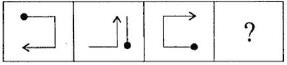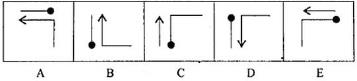Courses

# Olympiad Test : Analogy - 5

## 10 Questions MCQ Test Maths Olympiad Class 6 | Olympiad Test : Analogy - 5

Description
This mock test of Olympiad Test : Analogy - 5 for Class 6 helps you for every Class 6 entrance exam. This contains 10 Multiple Choice Questions for Class 6 Olympiad Test : Analogy - 5 (mcq) to study with solutions a complete question bank. The solved questions answers in this Olympiad Test : Analogy - 5 quiz give you a good mix of easy questions and tough questions. Class 6 students definitely take this Olympiad Test : Analogy - 5 exercise for a better result in the exam. You can find other Olympiad Test : Analogy - 5 extra questions, long questions & short questions for Class 6 on EduRev as well by searching above.
QUESTION: 1

### The second figure in the first unit of problem figures bears a certain relationship to the first figure. Similarly, one of the figures in the answer figures bears the same relationship to the first figure in the second unit of the problem figures. You are therefore to locate the figure which would fit the question mark. Problem Figures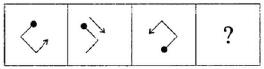Answer Figures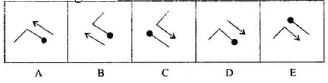Solution:

Black dot should be in right corner with opposite direction of arrow and arrow should be under the black dot.

QUESTION: 2

### The second figure in the first unit of problem figures bears a certain relationship to the first figure. Similarly, one of the figures in the answer figures bears the same relationship to the first figure in the second unit of the problem figures. You are therefore to locate the figure which would fit the question mark. Problem Figures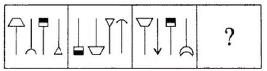Answer Figures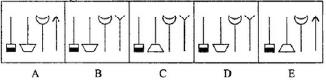Solution:

Third image should be in first place and gets inverted. First image should be in second place and gets inverted. Second image should be in fourth place and only outer part be inverted. We have to follow same pattern with fourth image.

QUESTION: 3

### The second figure in the first unit of problem figures bears a certain relationship to the first figure. Similarly, one of the figures in the answer figures bears the same relationship to the first figure in the second unit of the problem figures. You are therefore to locate the figure which would fit the question mark. Problem Figures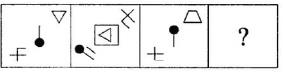Answer Figures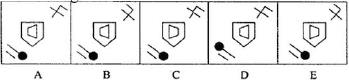Solution:

As per pattern trapezium should be in middle inside the pentagon with ninety degree rotation. Similar patter

QUESTION: 4

The second figure in the first unit of problem figures bears a certain relationship to the first figure. Similarly, one of the figures in the answer figures bears the same relationship to the first figure in the second unit of the problem figures. You are therefore to locate the figure which would fit the question mark.
Problem Figures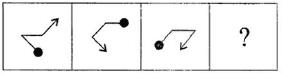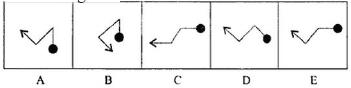Solution:

Arrow should be in right corner with upward direction. Line with black dot rotates through 45° ACW.

QUESTION: 5

The second figure in the first unit of problem figures bears a certain relationship to the first figure. Similarly, one of the figures in the answer figures bears the same relationship to the first figure in the second unit of the problem figures. You are therefore to locate the figure which would fit the question mark.
Problem Figures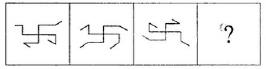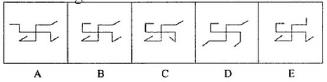Solution:

All the outer parts gets inside and inner part gets outside.

QUESTION: 6

The second figure in the first unit of problem figures bears a certain relationship to the first figure. Similarly, one of the figures in the answer figures bears the same relationship to the first figure in the second unit of the problem figures. You are therefore to locate the figure which would fit the question mark.
Problem Figures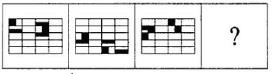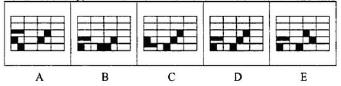Solution:

Shifting the shaded portion in opposite direction, we get the required image.

QUESTION: 7

The second figure in the first unit of problem figures bears a certain relationship to the first figure. Similarly, one of the figures in the answer figures bears the same relationship to the first figure in the second unit of the problem figures. You are therefore to locate the figure which would fit the question mark.
Problem Figures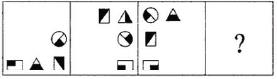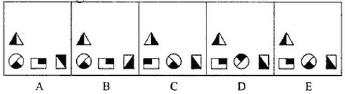Solution:

All the figures get inverted.

QUESTION: 8

The second figure in the first unit of problem figures bears a certain relationship to the first figure. Similarly, one of the figures in the answer figures bears the same relationship to the first figure in the second unit of the problem figures. You are therefore to locate the figure which would fit the question mark.
Problem Figures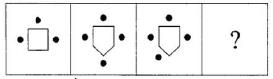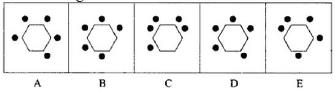Solution:

The number of dots is one less than the number of sides.

QUESTION: 9

The second figure in the first unit of problem figures bears a certain relationship to the first figure. Similarly, one of the figures in the answer figures bears the same relationship to the first figure in the second unit of the problem figures. You are therefore to locate the figure which would fit the question mark.
Problem Figures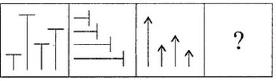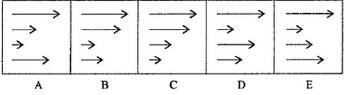Solution:

The number of positions of arrow are changed in order.

QUESTION: 10

The second figure in the first unit of problem figures bears a certain relationship to the first figure. Similarly, one of the figures in the answer figures bears the same relationship to the first figure in the second unit of the problem figures. You are therefore to locate the figure which would fit the question mark.
Problem Figures